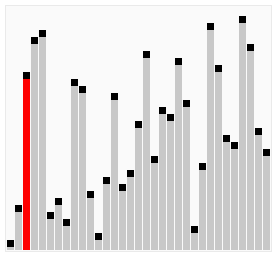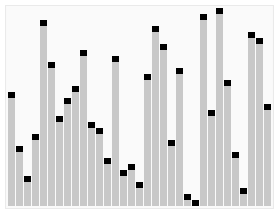01-02-2018

Bubble sort is a not very efficient algorithm and generally not used in real world applications. It is the simplest sorting algorithm It works by iterating over the array of values and swapping them if they are in the wrong order. Hence they ‘bubble up’ to their proper positions.

The simplest version will always run in O(n^2) time, even if the array is already sorted. An optimized version will stop once the inner loop didn’t swap once.A bubble sort algorithm

Time Complexity:

• Best Case: O(n)
• Worst Case: O(n^2)
• Average: O(n^2)
```              ```
n = 7
a = [4,3,8,6,1,2,6]

swaps = 0
for x in range(n):
currentSwaps = 0
for z in range(0, n-1):
if a[z] > a[z+1]:
a[z], a[z + 1] = a[z + 1], a[z]
swaps += 1
currentSwaps += 1
if currentSwaps = 0
break
```
```

### Quicksort

This is a very efficient sorting algorithm. It was developed by a British Computer Scientist Tony Hoare in 1959 and published in 1961.

It is a “Divide and Congquer” algorithm like Merge Sort. It picks an element as a pivot and partitions the array around it. It puts on one side all elements lower and on the other side all those that are higher.

There are different versions of the algorithm that pick the first pivot in different ways. Either as the first, last, random or median element in the array.

Time Complexity:

• Best Case: O(n log n)
• Worst Case: O(n^2)
• Average: O(n log n)A quicksort algorithm

```                ```
def partition(arr,low,high):
i = ( low-1 )         # index of smaller element
pivot = arr[high]     # pivot

for j in range(low , high):
if   arr[j] <= pivot:
i = i+1
arr[i],arr[j] = arr[j],arr[i]
arr[i+1],arr[high] = arr[high],arr[i+1]
return ( i+1 )

def quicksort(arr,low,high):
if low < high:
pi = partition(arr,low,high)
quicksort(arr, low, pi-1)
quicksort(arr, pi+1, high)
```
```